# How to divide in Excel and handle #DIV/0! error

The tutorial shows how to use a division formula in Excel to divide numbers, cells or entire columns and how to handle Div/0 errors.

As with other basic math operations, Microsoft Excel provides several ways to divide numbers and cells. Which one to use depends on your personal preferences and a particular task you need to solve. In this tutorial, you will find some good examples of using a division formula in Excel that cover the most common scenarios.

## Divide symbol in Excel

The common way to do division is by using the divide sign. In mathematics, the operation of division is represented by an obelus symbol (÷). In Microsoft Excel, the divide symbol is a forward slash (/).

With this approach, you simply write an expression like =a/b with no spaces, where:

• a is the dividend - a number you want to divide, and
• b is the divisor - a number by which the dividend is to be divided.

### How to divide numbers in Excel

To divide two numbers in Excel, you type the equals sign (=) in a cell, then type the number to be divided, followed by a forward slash, followed by the number to divide by, and press the Enter key to calculate the formula.

For example, to divide 10 by 5, you type the following expression in a cell: `=10/5`

The screenshot below shows a few more examples of a simple division formula in Excel: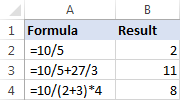When a formula performs more than one arithmetic operation, it is important to remember about the order of calculations in Excel (PEMDAS): parentheses first, followed by exponentiation (raising to power), followed by multiplication or division whichever comes first, followed by addition or subtraction whichever comes first.

### How to divide a cell in Excel

To divide two cells in Excel, you use the divide symbol exactly like shown in the above examples, but supply cell references instead of numbers.

For example:

• To divide cell A2 by 5: `=A2/5`
• To divide cell A2 by cell B2: `=A2/B2`
• To divide multiple cells successively, type cell references separated by the division symbol. For example, to divide the number in A2 by the number in B2, and then divide the result by the number in C2, use this formula: `=A2/B2/C2`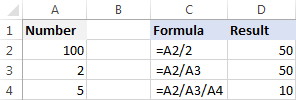## Divide function in Excel (QUOTIENT)

I have to say plainly: there is no Divide function in Excel. Whenever you want to divide one number by another, use the division symbol as explained in the above examples.

However, if you want to return only the integer portion of a division and discard the remainder, then use the QUOTIENT function:

QUOTIENT(numerator, denominator)

Where:

• Numerator (required) - the dividend, i.e. the number to be divided.
• Denominator (required) - the divisor, i.e. the number to divide by.

When two numbers divide evenly without remainder, the division symbol and a QUOTIENT formula return the same result. For example, both `=10/5` and `=QUOTIENT(10, 5)` return 2.

When there is a remainder after division, the divide sign returns a decimal number and the QUOTIENT function returns only the integer part. For example, `=5/4` returns 1.25 and `=QUOTIENT(5,4)` yields 1.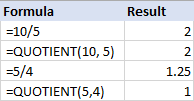### 3 things you should know about QUOTIENT function

As simple as it seems, the Excel QUOTIENT function still has a few caveats you should be aware of:

1. The numerator and denominator arguments should be supplied as numbers, references to cells containing numbers, or other functions that return numbers.
2. If either argument is non-numeric, a QUOTIENT formula returns the #VALUE! error.
3. If denominator is 0, QUOTIENT returns the divide by zero error (#DIV/0!).

## How to divide columns in Excel

Dividing columns in Excel is also easy. It can done by copying a regular division formula down the column or by using an array formula. Why would one want to use an array formula for a trivial task like that? You will learn the reason in a moment :)

### How to divide two columns in Excel by copying a formula

To divide columns in Excel, just do the following:

1. Divide two cells in the topmost row, for example: `=A2/B2`
2. Insert the formula in the first cell (say C2) and double-click the small green square in the lower-right corner of the cell to copy the formula down the column. Done!

Since we use relative cell references (without the \$ sign), our division formula will change based on a relative position of a cell where it is copied: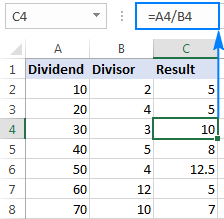Tip. In a similar fashion, you can divide two rows in Excel. For example, to divide values in row 1 by values in row 2, you put `=A1/A2` in cell A3, and then copy the formula rightwards to as many cells as necessary.

### How to divide one column by another with an array formula

In situations when you want to prevent accidental deletion or alteration of a formula in individual cells, insert an array formula in an entire range.

For example, to divide the values in cells A2:A8 by the values in B2:B8 row-by-row, use this formula: `=A2:A8/B2:B8`

To insert the array formula correctly, perform these steps:

1. Select the entire range where you want to enter the formula (C2:C8 in this example).
2. Type the formula in the formula bar and press Ctrl + Shift + Enter to complete it. As soon as you do this, Excel will enclose the formula in {curly braces}, indicating it's an array formula.

As the result, you will have the numbers in column A divided by the numbers in column B in one fell swoop. If someone tries to edit your formula in an individual cell, Excel will show a warning that part of an array cannot be changed.

To delete or modify the formula, you will need to select the whole range first, and then make the changes. To extend the formula to new rows, select the entire range including new rows, change the cell references in the formula bar to accommodate new cells, and then press Ctrl + Shift + Enter to update the formula.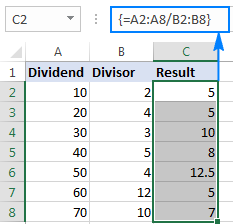## How to divide a column by a number in Excel

Depending on whether you want the output to be formulas or values, you can divide a column of numbers by a constant number by using a division formula or Paste Special feature.

### Divide a column by number with a formula

As you already know, the fastest way to do division in Excel is by using the divide symbol. So, to divide each number in a given column by the same number, you put a usual division formula in the first cell, and then copy the formula down the column. That's all there is to it!

For example, to divide values in column A by the number 5, insert the following formula in A2, and then copy it down to as many cells as you want: `=A2/5`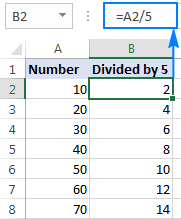As explained in the above example, the use of a relative cell reference (A2) ensures that the formula gets adjusted properly for each row. That is, the formula in B3 becomes `=A3/5`, the formula in B4 becomes `=A4/5`, and so on.

Instead of supplying the divisor directly in the formula, you can enter it in some cell, say D2, and divide by that cell. In this case, it's important that you lock the cell reference with the dollar sign (like \$D\$2), making it an absolute reference because this reference should remain constant no matter where the formula is copied.

As shown in the screenshot below, the formula `=A2/\$D\$2` returns exactly the same results as `=A2/5`.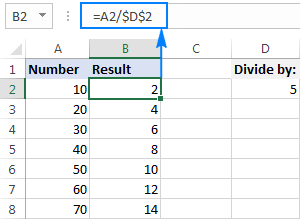### Divide a column by the same number with Paste Special

In case you want the results to be values, not formulas, you can do division in the usual way, and then replace formulas with values. Or, you can achieve the same result faster with the Paste Special > Divide option.

1. If you don't want to override the original numbers, copy them to the column where you want to have the results. In this example, we copy numbers from column A to column B.
2. Put the divisor in some cell, say D2, like shown in the screenshot below.
3. Select the divisor cell (D5), and press Ctrl + C to copy it to the clipboard.
4. Select the cells you want to multiply (B2:B8).
5. Press Ctrl + Alt + V, then I, which is the shortcut for Paste Special > Divide, and hit the Enter key.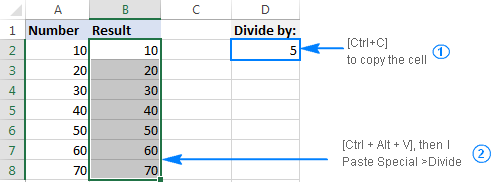Alternatively, right-click the selected numbers, select Paste Special… from the context menu, then select Divide under Operation, and click OK.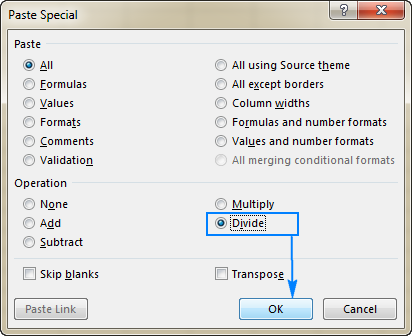Either way, each of the selected numbers in column A will be divided by the number in D5, and the results will be returned as values, not formulas: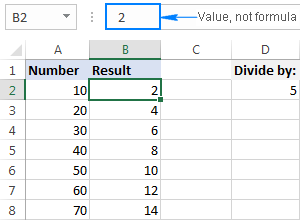## How to divide by percentage in Excel

Since percentages are parts of larger whole things, some people think that to calculate percentage of a given number you should divide that number by percent. But that is a common delusion! To find percentages, you should multiply, not divide. For example, to find 20% of 80, you multiply 80 by 20% and get 16 as the result: 80*20%=16 or 80*0.2=16.

In what situations do you divide a number by percentage? For instance, to find X if a certain percent of X is Y. To make things clearer, let's solve this problem: 100 is 25% of what number?

To get the answer, convert the problem to this simple equation:

X = Y/P%

With Y equal to 100 and P to 25%, the formula takes the following shape: `=100/25%`

Since 25% is 25 parts of a hundred, you can safely replace the percentage with a decimal number: `=100/0.25`

As shown in the screenshot below, the result of both formulas is 400: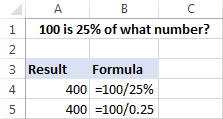For more examples of percentage formulas, please see How to calculate percentages in Excel.

## Excel DIV/0 error

Division by zero is an operation for which there exists no answer, therefore it is disallowed. Whenever you try to divide a number by 0 or by an empty cell in Excel, you will get the divide by zero error (#DIV/0!). In some situations, that error indication might be useful, alerting you about possible faults in your data set.

In other scenarios, your formulas can just be waiting for input, so you may wish to replace Excel Div 0 error notations with empty cells or with your own message. That can be done by using either an IF formula or IFERROR function.

### Suppress #DIV/0 error with IFERROR

The easiest way to handle the #DIV/0! error in Excel is to wrap your division formula in the IFERROR function like this:

`=IFERROR(A2/B2, "")`

The formula checks the result of the division, and if it evaluates to an error, returns an empty string (""), the result of the division otherwise.

Please have a look at the two worksheets below. Which one is more aesthetically pleasing?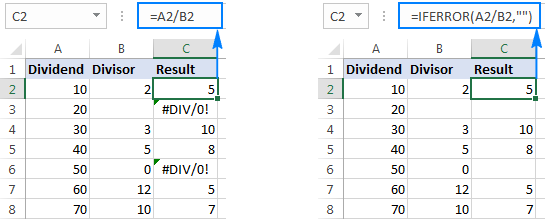Note. Excel's IFERROR function disguises not only #DIV/0! errors, but all other error types such as #N/A, #NAME?, #REF!, #VALUE!, etc. If you'd like to suppress specifically DIV/0 errors, then use an IF formula as shown in the next example.

### Handle Excel DIV/0 error with IF formula

To mask only Div/0 errors in Excel, use an IF formula that checks if the divisor is equal (or not equal) to zero.

For example:

`=IF(B2=0,"",A2/B2)`

Or

`=IF(B2<>0,A2/B2,"")`

If the divisor is any number other than zero, the formulas divide cell A2 by B2. If B2 is 0 or blank, the formulas return nothing (empty string).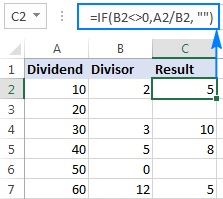Instead of an empty cell, you could also display a custom message like this:

`=IF(B2<>0, A2/B2, "Error in calculation")`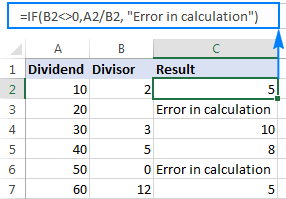## How to divide with Ultimate Suite for Excel

If you are making your very first steps in Excel and do not feel comfortable with formulas yet, you can do division by using a mouse. All it takes is our Ultimate Suite installed in your Excel.

In one of the examples discussed earlier, we divided a column by a number with Excel's Paste Special. That involved a lot of mouse movement and two shortcuts. Now, let me show you a shorter way to do the same.

1. Copy the numbers you want to divide in the "Results" column to prevent the original numbers from being overridden.
2. Select the copied values (C2:C5 in the screenshot below).
3. Go to the Ablebits tools tab > Calculate group, and do the following:
• Select the divide sign (/) in the Operation box.
• Type the number to divide by in the Value box.
• Click the Calculate button.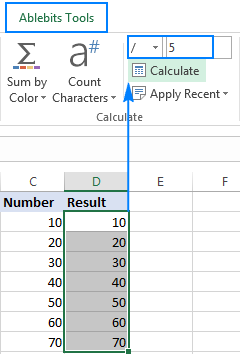Done! The entire column is divided by the specified number in the blink of an eye: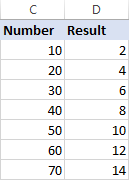As with Excel Paste Special, the result of division is values, not formulas. So, you can safely move or copy the output to another location without worrying about updating formula references. You can even move or delete the original numbers, and your calculated numbers will be still safe and sound.

That is how you divide in Excel by using formulas or Calculate tools. If are curious to try this and many other useful features included with the Ultimate Suite for Excel, you are welcome to download 14-day trial version.

To have a closer look at the formulas discussed in this tutorial, feel free to download our Excel Division formula examples.

I thank you for reading and hope to see you on our blog next week!

### 24 responses to "How to divide in Excel and handle #DIV/0! error"

1.arpa says:

=SUM(BO3,BP3,BR3)-MIN(BO3,BP3,BR3)

i want to divide it by 2

•Doug says:

Arpa:
Try =SUM((BO3,BP3,BR3)-MIN(BO3,BP3,BR3))/2

2.viji says:

=(A1-B2)/B2 the answer should be in %
for ex
(10-0)/0 =100%

•Kishan says:

Click on Percent Style under Number group in Home Tab. 2nd way that Press (Ctrl+Shift+%)

3.nadir says:

951 - 10 3639769 #VALUE!

4.prudhvi says:

please be note that i want to divide one column and drag to divide all the column with same formula please help me now

5.pushpa shahi says:

formula of division in excel

6.liu xiaoyun says:

quite useful , thank you

7.Karen says:

The "Divide a column by the same number with Paste Special" was extremely helpful, thank you!

8.true says:

Good. Thank you.

•Vry true says:

Most Welcome

9.Raj Sharma says:

=E5/E6
Iska write formula kya hoga sir

10.rakesh says:

how to use divided formula in name and amount please said the formula

11.mushtaque says:

How to multiply (1.84+1.86)/2*(1.46+1.44)/2 in excel

12.Gilmer says:

How will i divide a number and put the quotient into multiple cells 3 to four cells, quotient should be a whole number.

13.Stefan says:

Hi,
Can someone explain to me how can I divide one number equally into more cells?
This is to do with eBay sales. I have 25 items and I want to create different listings like people having the option to buy 1, 2, 3, 5, and I want to divide the 25 in like (2x5=10) remaining 15 (2x3=6) remaining 9 3x2=6 remaining 3x1. I hope it makes sense.

Thank you.

14.Ivan says:

I'm trying to divide my social media as follows, I want to get the reach and divide it by my the sum of comments, likes, and shares.

So ideally the formula would be like this, if my reach is 1000 and my comments are 15, likes are 5, and shares are 15 I would like for these to be added together and then have the 1000 value divided by that sum.

Hope that makes sense

•Alexander Trifuntov (Ablebits.com Team) says:

Hello Ivan!

=IF(AND(A1>=1000,B1>=15,C1>=5,D1>=15),1000/(A1+B1+C1+D1),0)

Hope this is what you need.

15.Mehr says:

Formula to add the contents of B1 to B5 and divide the sum by contents of C7.

•Alexander Trifuntov (Ablebits.com Team) says:

Hello!

=SUM(B1:B5)/C7

16.Cody says:

Is there a way to divide a set of numbers by the amount of data points selected? For example if I put data into 5 different cells I want a formula that will add those 5 numbers together then divide by 5 for an average. If I were to add another data point then it would add the 6 numbers together and divide by 6. Or do I just have to do this manually? TIA

•Alexander Trifuntov (Ablebits.com Team) says:

Hello Cody!
I recommend making your data into a table. The table will automatically resize when you add data. Read here how to do it.
You can then use the table data in formulas as described in this article.

17.John says: